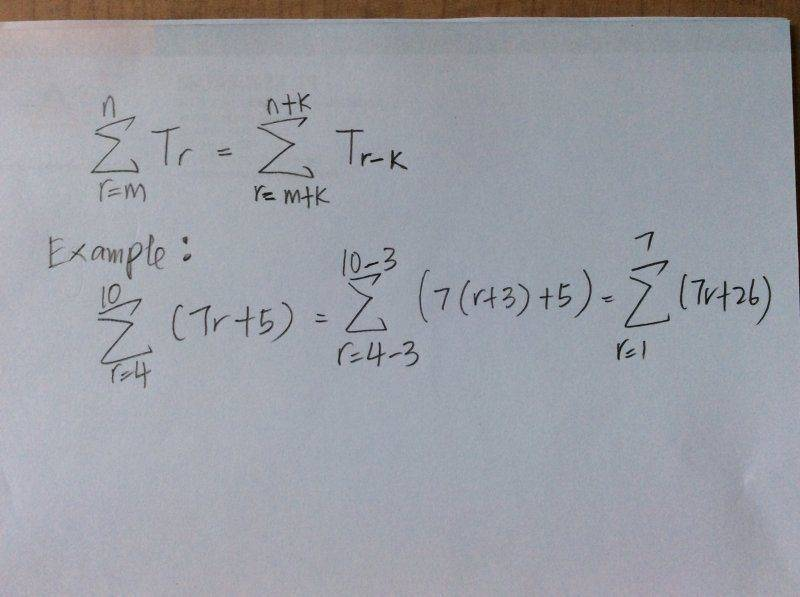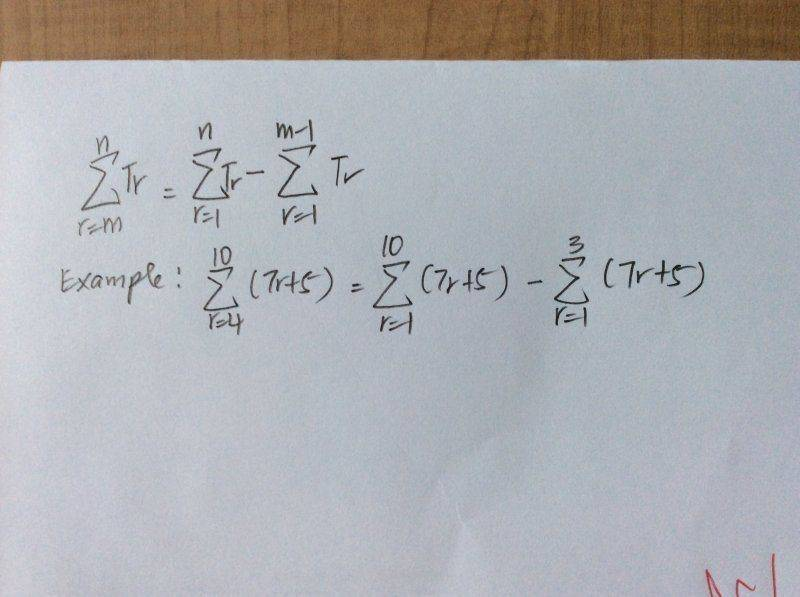# Changing the Limits of Summation

• LiHJ
In summary, the homework equations are:-The sum of a sequence is the sum of the individual terms.-If one variable is changed, the sum is unchanged.

## Homework Statement

Dear Mentors and PF helpers,

Here's my question, I see these on my textbook but couldn't really understand how they derived this short cut.
Please show me how they got to these. Thank you for your time.## Homework Equations

These is what I understand from now.## The Attempt at a Solution

So we have the sum $\displaystyle \sum_{r=m}^n T_r$. We may change the index of summation from r to s=r+k. Then because r starts from m, s should start from m+k and because r ends at n, s should end at n+k. This means that we changed our summation to $\displaystyle \sum_{s=m+k}^{n+k}T_{s-k}$. But you should note that this sum doesn't depend on s as the first sum didn't depend on r. r and s are just dummy indices because after you do the sums, there'll remain no sign of them. So I can safely rename s to r. But because the sums are just numbers and because the changes I did to the first sum doesn't change the result, I'll have:
$\displaystyle \sum_{r=m}^n T_r=\sum_{r=m+k}^{n+k}T_{r-k}$.

Dear Shyan,

Do you mind giving me a better view with these words, can give your explanation with examples to explain these.

$\displaystyle \sum_{r=m}^n T_r=T_m+T_{m+1}+T_{m+2}+\dots+T_{n-2}+T_{n-1}+T_n$
$\displaystyle \sum_{r=m+k}^{n+k} T_{r-k}=T_{m+k-k}+T_{m+k+1-k}+T_{m+k+2-k}+\dots+T_{n+k-2-k}+T_{n+k-1-k}+T_{n+k-k}=\\ T_m+T_{m+1}+T_{m+2}+\dots+T_{n-2}+T_{n-1}+T_n$